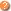# Programming FAQHow to create an invisible DataMatrix ActiveX in Visual Basic?How to print a DataMatrix barcode from Visual Basic?

# How to create an invisible DataMatrix ActiveX in Visual Basic?

For the Barcode ActiveX control to be used, there is no need to place it on a form as shown here. You can create an invisible DataMatrix barcode control from any application including your DLL.
The first thing needed in order to do it is to create an object using the CreateObject function (as shown below). After the object is created, you can use it as you want.
'Create a new instance of DataMatrix-ActiveX
Dim oDataMatrix As Object
Set oDataMatrix = CreateObject("DataMatrixAx.DataMatrixCtrl.1")

'set DataMatrix-ActiveX properties
oDataMatrix.DataToEncode = "Hello world"

'using DataMatrix-ActiveX
Call oDataMatrix.DrawMatrixToSize(5, 5, 100, 100, dmPixels, Form1.hdc)

'destroy DataMatrix-ActiveX object
Set oDataMatrix = Nothing

# How to print a DataMatrix barcode from Visual Basic?

There are a few different ways to print a barcode from Visual Basic using our ActiveX Control.
1. Using a Visual Basic Printer object.

Printer.CurrentX = 2048
Printer.Print "BarcodeTools.com, VB Example"

'DataMatrix-ActiveX will use the Visual Basic Printer object
Call DataMatrixCtrl1.SetPrinterHDC(Printer.hdc)

'print two barcodes
Call DataMatrixCtrl1.DrawMatrixToSize(10, 10, 30, 30, dmMM)
Call DataMatrixCtrl1.DrawMatrixToSize(70, 10, 30, 30, dmMM)

Printer.EndDoc

2. Using DataMatrix-ActiveX methods.

'open a current printer
Call DataMatrixCtrl1.BeginPrint("")

'print two DataMatrix barcodes
Call DataMatrixCtrl1.DrawMatrixToSize(10, 10, 30, 30, dmMM)
Call DataMatrixCtrl1.DrawMatrixToSize(70, 10, 30, 30, dmMM)

Call DataMatrixCtrl1.EndPrint

3. How to print a DataMatrix barcode with a certain x-dimension?.

To find out what x-dimension is, click here.
To print a barcode with a certain x-dimension, you should correctly specify the barcode width. You can calculate it using the GetMatrixSize method.

```Private Declare Function GetDeviceCaps Lib "gdi32" _
(ByVal hdc As Long, ByVal nIndex As Long) As Long

Private Const LOGPIXELSX = 88
Private Const LOGPIXELSY = 90
Private Sub PrintBarcode()
'Create a new instance of DataMatrix-ActiveX
Dim oDataMatrix As Object
Set oDataMatrix = CreateObject("DataMatrixAx.DataMatrixCtrl.1")
'set DataMatrix-ActiveX properties
oDataMatrix.DataToEncode = "Hello world"
oDataMatrix.PreferredFormat = 0
'open a current printer
Call oDataMatrix.BeginPrint("")

'gets the printer resolution (dpi - dots per inch)
Dim dpiX As Long, dpiY As Long
dpiX = GetDeviceCaps(Printer.hdc, LOGPIXELSX)
dpiY = GetDeviceCaps(Printer.hdc, LOGPIXELSY)
'calculate the required DataMatrix size (in mm) for the
'x-dimension of 0.508 mm (20 Mils)
Dim dmWidth, dmHeight
Call oDataMatrix.GetMatrixSize(0.508, dpiX, dpiY, dmMM, dmMM, _
dmWidth, dmHeight)
'print a barcode
Call oDataMatrix.DrawMatrixToSize(10, 10, dmWidth, dmHeight, dmMM, 0)
Call oDataMatrix.EndPrint
Set oDataMatrix = Nothing
End Sub
```